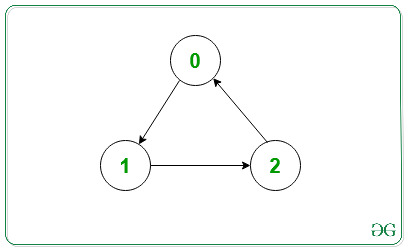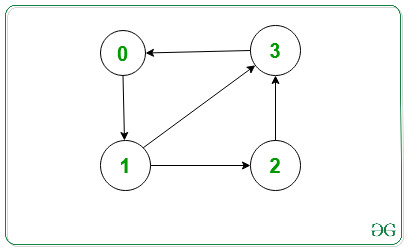# Print Adjacency List for a Directed Graph

• Last Updated : 11 Feb, 2022

An Adjacency List is used for representing graphs. Here, for every vertex in the graph, we have a list of all the other vertices which the particular vertex has an edge to.

Problem: Given the adjacency list and number of vertices and edges of a graph, the task is to represent the adjacency list for a directed graph.

Examples:

Input: V = 3, edges[][]= {{0, 1}, {1, 2} {2, 0}}Output:   0 -> 1
1 -> 2
2 -> 0
Explanation:
The output represents the adjacency list for the given graph.

Input: V = 4, edges[][] = {{0, 1}, {1, 2}, {1, 3}, {2, 3}, {3, 0}}Output:   0 -> 1
1 -> 2 3
2 -> 3
3 -> 0
Explanation:
The output represents the adjacency list for the given graph.

Approach (using STL): The main idea is to represent the graph as an array of vectors such that every vector represents the adjacency list of a single vertex. Using STL, the code becomes simpler and easier to understand.

Below is the implementation of the above approach:

## C++

 `// C++ program for the above approach`   `#include ` `using` `namespace` `std;`   `// Function to add edges` `void` `addEdge(vector<``int``> adj[], ``int` `u, ``int` `v)` `{` `    ``adj[u].push_back(v);` `}`   `// Function to print adjacency list` `void` `adjacencylist(vector<``int``> adj[], ``int` `V)` `{` `    ``for` `(``int` `i = 0; i < V; i++) {` `        ``cout << i << ``"->"``;` `        ``for` `(``int``& x : adj[i]) {` `            ``cout << x << ``" "``;` `        ``}` `        ``cout << endl;` `    ``}` `}`   `// Function to initialize the adjacency list` `// of the given graph` `void` `initGraph(``int` `V, ``int` `edges, ``int` `noOfEdges)` `{` `    ``// To represent graph as adjacency list` `    ``vector<``int``> adj[V];`   `    ``// Traverse edges array and make edges` `    ``for` `(``int` `i = 0; i < noOfEdges; i++) {`   `        ``// Function call to make an edge` `        ``addEdge(adj, edges[i], edges[i]);` `    ``}`   `    ``// Function Call to print adjacency list` `    ``adjacencylist(adj, V);` `}`   `// Driver Code` `int` `main()` `{` `    ``// Given vertices` `    ``int` `V = 3;`   `    ``// Given edges` `    ``int` `edges = { { 0, 1 }, { 1, 2 }, { 2, 0 } };`   `    ``int` `noOfEdges = 3;`   `    ``// Function Call` `    ``initGraph(V, edges, noOfEdges);`   `    ``return` `0;` `}`

## Java

 `// Java program for the above approach` `import` `java.util.*;`   `class` `GFG` `{`   `  ``// Function to add edges` `  ``static` `void` `addEdge(Vector adj[], ``int` `u, ``int` `v)` `  ``{` `    ``adj[u].add(v);` `  ``}`   `  ``// Function to print adjacency list` `  ``static` `void` `adjacencylist(Vector adj[], ``int` `V)` `  ``{` `    ``for` `(``int` `i = ``0``; i < V; i++) {` `      ``System.out.print(i+ ``"->"``);` `      ``for` `(``int` `x : adj[i]) {` `        ``System.out.print(x+ ``" "``);` `      ``}` `      ``System.out.println();` `    ``}` `  ``}`   `  ``// Function to initialize the adjacency list` `  ``// of the given graph` `  ``static` `void` `initGraph(``int` `V, ``int` `edges[][], ``int` `noOfEdges)` `  ``{`   `    ``// To represent graph as adjacency list` `    ``@SuppressWarnings``(``"unchecked"``)` `    ``Vector adj[] = ``new` `Vector[``3``];` `    ``for``(``int` `i =``0``;i();` `    ``}` `    ``// Traverse edges array and make edges` `    ``for` `(``int` `i = ``0``; i < noOfEdges; i++) {`   `      ``// Function call to make an edge` `      ``addEdge(adj, edges[i][``0``], edges[i][``1``]);` `    ``}`   `    ``// Function Call to print adjacency list` `    ``adjacencylist(adj, V);` `  ``}`   `  ``// Driver Code` `  ``public` `static` `void` `main(String[] args)` `  ``{` `    ``// Given vertices` `    ``int` `V = ``3``;`   `    ``// Given edges` `    ``int` `edges[][] = { { ``0``, ``1` `}, { ``1``, ``2` `}, { ``2``, ``0` `} };`   `    ``int` `noOfEdges = ``3``;`   `    ``// Function Call` `    ``initGraph(V, edges, noOfEdges);`   `  ``}` `}`   `// This code is contributed by gauravrajput1 `

## Python3

 `# Python program for the above approach`   `# Function to add edges` `def` `addEdge(adj, u, v):` `    ``adj[u].append(v)` `  `  `# Function to print adjacency list` `def` `adjacencylist(adj, V):` `    `  `    ``for` `i ``in` `range` `(``0``, V):` `        ``print``(i, ``"->"``, end``=``"")` `        `  `        ``for` `x ``in`  `adj[i]: ` `            ``print``(x , ``" "``, end``=``"")` `      `  `        ``print``()` `    `  `# Function to initialize the adjacency list` `# of the given graph` `def` `initGraph(V, edges, noOfEdges):`   `    ``adj ``=` `[``0``]``*` `3` `    `  `    ``for` `i ``in` `range``(``0``, ``len``(adj)):` `        ``adj[i] ``=` `[]` `  `  `    ``# Traverse edges array and make edges` `    ``for` `i ``in` `range``(``0``, noOfEdges) :`   `         ``# Function call to make an edge` `        ``addEdge(adj, edges[i][``0``], edges[i][``1``])` `    `    `    ``# Function Call to print adjacency list` `    ``adjacencylist(adj, V)` `  `  `# Driver Code` `  `  `# Given vertices` `V ``=` `3`   `# Given edges` `edges ``=`  `[[``0``, ``1` `],  [``1``, ``2` `],  [``2``, ``0` `]] `   `noOfEdges ``=` `3``;`   `# Function Call` `initGraph(V, edges, noOfEdges)`   `# This code is contributed by AR_Gaurav`

## C#

 `// C# program for the above approach` `using` `System;` `using` `System.Collections.Generic;`   `public` `class` `GFG` `{`   `  ``// Function to add edges` `  ``static` `void` `addEdge(List<``int``> []adj, ``int` `u, ``int` `v)` `  ``{` `    ``adj[u].Add(v);` `  ``}`   `  ``// Function to print adjacency list` `  ``static` `void` `adjacencylist(List<``int``> []adj, ``int` `V)` `  ``{` `    ``for` `(``int` `i = 0; i < V; i++) {` `      ``Console.Write(i+ ``"->"``);` `      ``foreach` `(``int` `x ``in` `adj[i]) {` `        ``Console.Write(x+ ``" "``);` `      ``}` `      ``Console.WriteLine();` `    ``}` `  ``}`   `  ``// Function to initialize the adjacency list` `  ``// of the given graph` `  ``static` `void` `initGraph(``int` `V, ``int` `[,]edges, ``int` `noOfEdges)` `  ``{`   `    ``// To represent graph as adjacency list   ` `    ``List<``int``> []adj = ``new` `List<``int``>;` `    ``for``(``int` `i = 0; i < adj.Length; i++) {` `      ``adj[i] = ``new` `List<``int``>();` `    ``}` `    `  `    ``// Traverse edges array and make edges` `    ``for` `(``int` `i = 0; i < noOfEdges; i++) {`   `      ``// Function call to make an edge` `      ``addEdge(adj, edges[i,0], edges[i,1]);` `    ``}`   `    ``// Function Call to print adjacency list` `    ``adjacencylist(adj, V);` `  ``}`   `  ``// Driver Code` `  ``public` `static` `void` `Main(String[] args)` `  ``{` `    ``// Given vertices` `    ``int` `V = 3;`   `    ``// Given edges` `    ``int` `[,]edges = { { 0, 1 }, { 1, 2 }, { 2, 0 } };`   `    ``int` `noOfEdges = 3;`   `    ``// Function Call` `    ``initGraph(V, edges, noOfEdges);`   `  ``}` `}`   `// This code is contributed by Amit Katiyar`

## Javascript

 ``

Output:

```0->1
1->2
2->0```

My Personal Notes arrow_drop_up
Recommended Articles
Page :